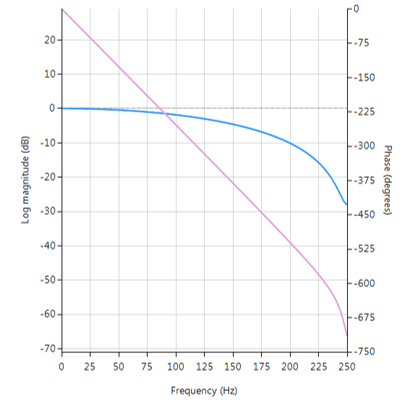# Fractional Farrow Delay Filter

In signal processing, the need sometimes arises to nudge or fine-tune the sampling instants of a signal by a fraction of a sample. An FIR Farrow delay filter is typically employed to achieve this task, and may be combined with a traditional integer delay line in order to achieve a universal fractional length delay line.

A Fractional delay based on an FIR Farrow structure may be defined as:

$$H(z)=(1-\alpha)+\alpha z^{-1}; \; 0 \leq \alpha \leq 1$$

Which produces a fractional linear delay of $$\alpha$$ between 0 and 1 samples. However, a more universal building block can be achieved by combining the Farrow delay structure with an integer delay, $$\Delta$$

$$H(z)=(1-\alpha) z^{-\Delta}+\alpha z^{-(\Delta+1)}$$

The plot shown below shows the magnitude (blue) and phase (purple) spectra for $$\Delta=9, \, \alpha=0.52$$. As seen, the fractional delay element results in a non-flat magnitude spectrum at higher frequencies.Frequency reponse of Farrow delay filter.

## Implementation

A Farrow delay filter may be implemented in ASN FilterScript as follows:

ClearH1;  // clear primary filter from cascade

interface alpha = {0,1,0.02,.5}; // fractional delay
interface D = {1,30,1,10};       // integer delay

Main()
Num = {zeros(D),1-alpha,alpha}; // numerator coefficients
Den = {1};                      // denominator coefficient
Gain = 1/sum(Num);              // normalise gain at DCTags:
0 replies